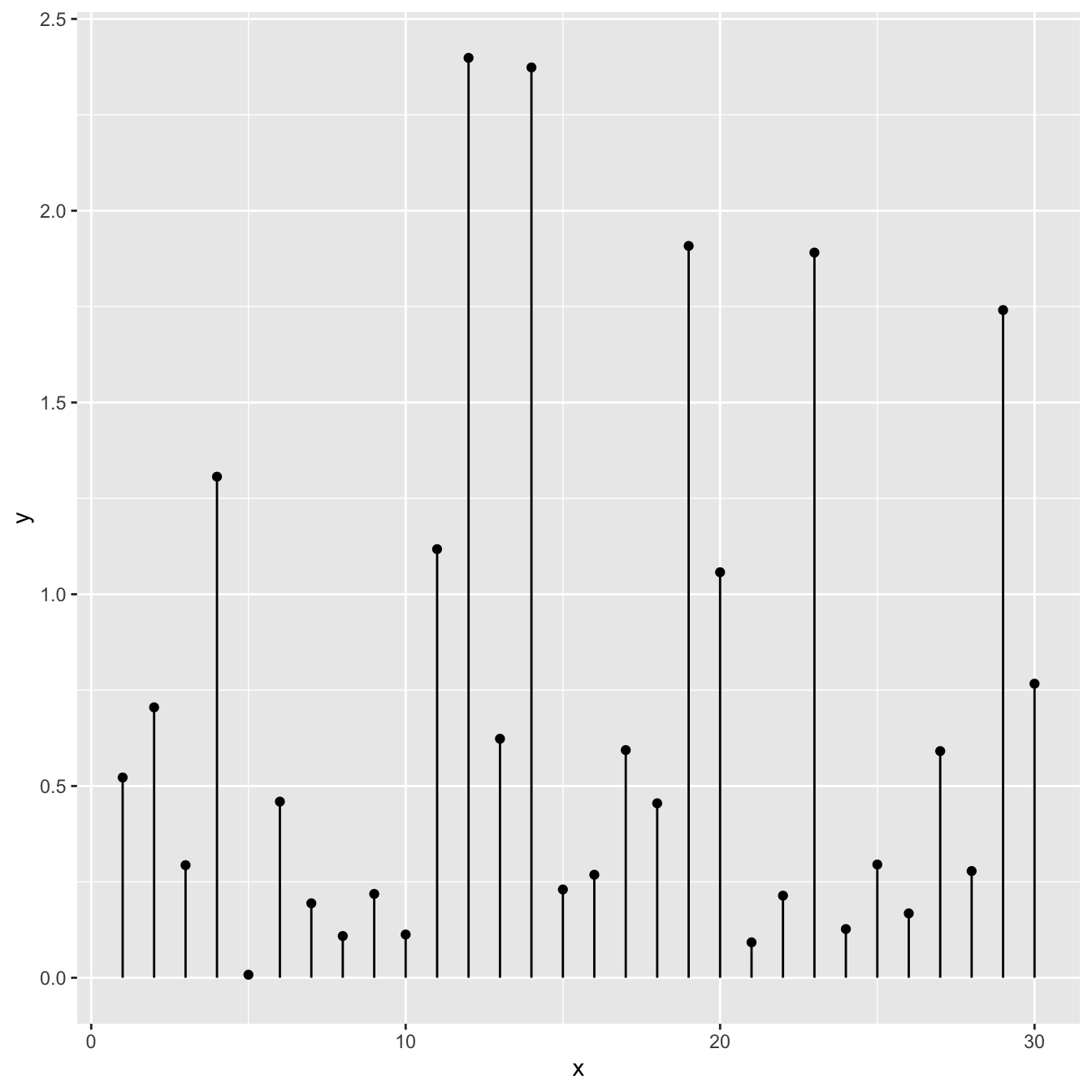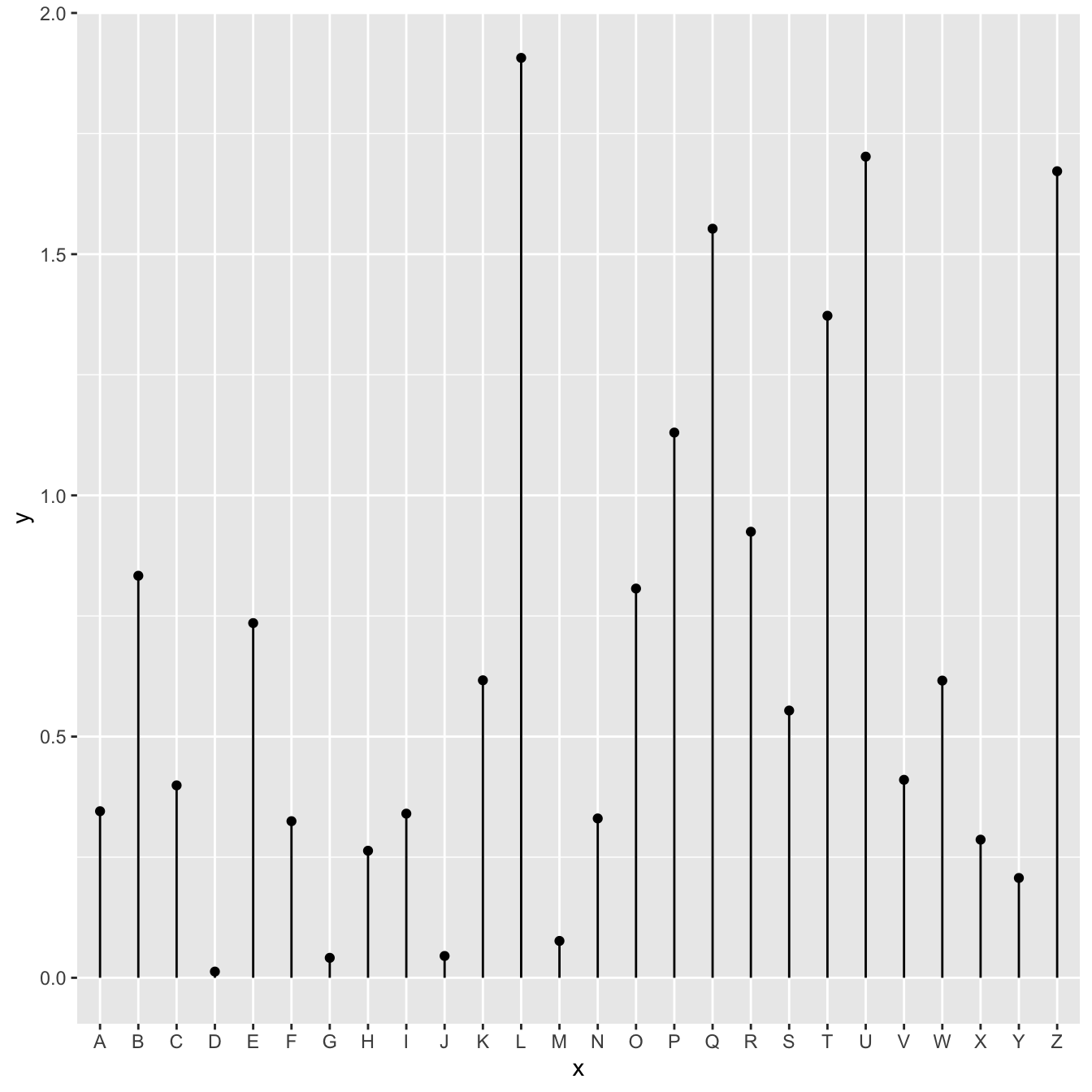# Most basic lollipop plot

How to build a very basic lollipop chart with `R` and ggplot2. This post how to use `geom_point()` and `geom_segment()` based on different input formats.

# From 2 numeric variables

A lollipop plot is very close from both scatterplots and barplots.

Thus, 2 types of input format work to build it:

• 2 numeric values like for a scatterplot
• one numeric and one categorical variable like for the barplot.

In any case, a lollipop is built using `geom_point()` for the circle, and `geom_segment()` for the stem.``````# Libraries
library(ggplot2)

# Create data
data <- data.frame(x=seq(1,30), y=abs(rnorm(30)))

# Plot
ggplot(data, aes(x=x, y=y)) +
geom_point() +
geom_segment( aes(x=x, xend=x, y=0, yend=y))``````

# From 1 numeric and 1 categorical variable

The code works pretty much the same way, but it is important to note that the X axis can represent a categorical variable as well. In this case, the lollipop chart is a good replacement of the barplot.``````# Libraries
library(ggplot2)

# Create data
data <- data.frame(
x=LETTERS[1:26],
y=abs(rnorm(26))
)

# Plot
ggplot(data, aes(x=x, y=y)) +
geom_point() +
geom_segment( aes(x=x, xend=x, y=0, yend=y))``````

# What’s next

The lollipop chart is one of my favourite. There is so much to do with it and it is under-utilized in favor of barplot. Visit the dedicated section for more examples produced with `R`, or data-to-viz to learn about the available variations and caveats to avoid.

Related chart types

## Contact

This document is a work by Yan Holtz. Any feedback is highly encouraged. You can fill an issue on Github, drop me a message on Twitter, or send an email pasting yan.holtz.data with gmail.com.